# Year 4 Maths Printable Worksheets

Help your child build on what they've already learned with our year 4 maths worksheets. Covering topics like times tables, properties of 2D and 3D shapes, estimating, measuring and recording results, fractions, decimals, mental maths and more, your child will find a variety of resources in our year 4 maths worksheets.

Filters

### Bar Modelling - 12 xtable

Bar models can be used to solve different kinds of multiplication. Students answer these 12 xtable questions as quickly as possible.

Age: 8 - 9
1
2
3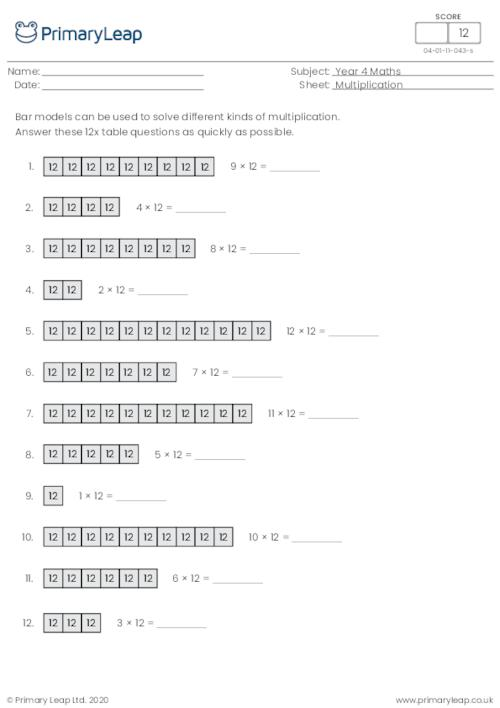### Bar Modelling - 6 xtable

Bar models can be used to solve different kinds of multiplication. Students answer these 6 xtable questions as quickly as possible.

Age: 8 - 9
1
2
3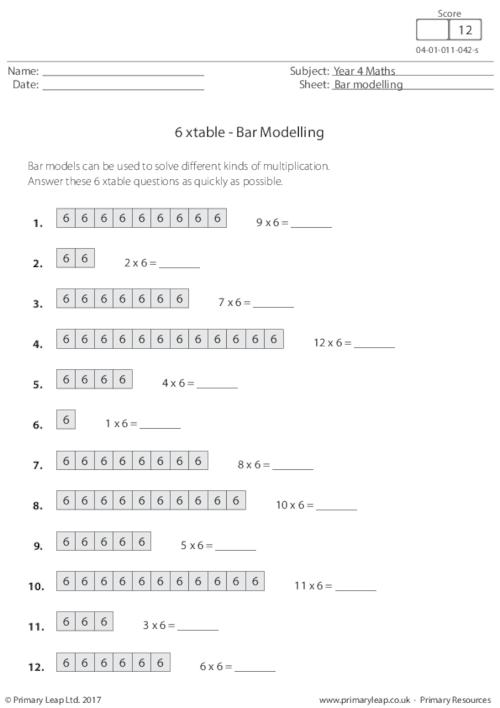### Bar Modelling - 7 xtable

Bar models can be used to solve different kinds of multiplication. Students answer these 7 xtable questions as quickly as possible.

Age: 8 - 9
1
2
3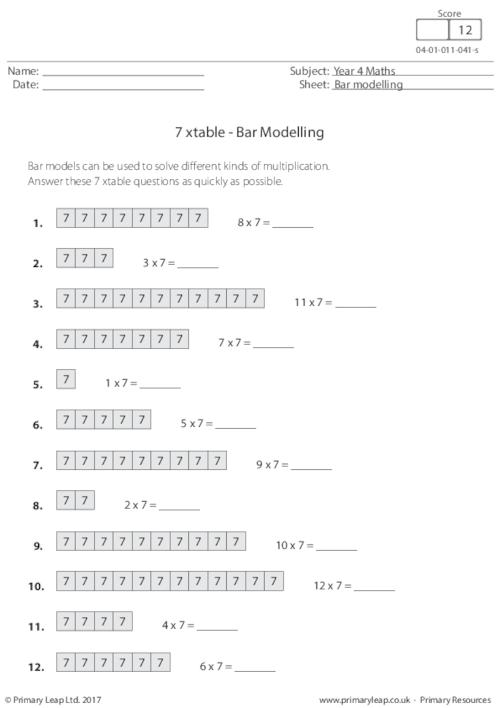### 1-Minute Maths - Multiplication (1)

This maths worksheet asks students to complete as many of the sums as they can in 1 minute. This will help a child's basic calculation skills and make more difficult maths easier for them later on.

Age: 8 - 9
1
2
3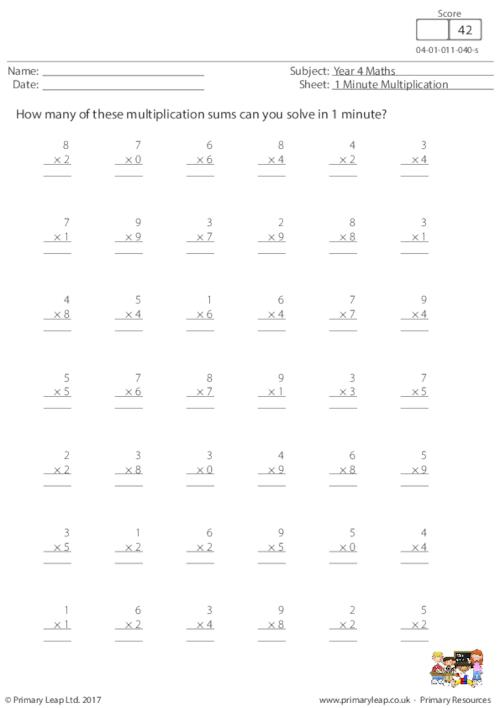### Lattice Multiplication: 2 by 2 digits (3)

Lattice multiplication is a method of multiplying numbers using a grid. This method breaks the multiplication process into smaller steps, which some students may find easier. There are 9 sums for students to work out.

Age: 8 - 9
1
2
3### Lattice Multiplication: 2 by 2 digits (2)

Lattice multiplication is a method of multiplying numbers using a grid. This method breaks the multiplication process into smaller steps, which some students may find easier.

Age: 8 - 9
1
2
3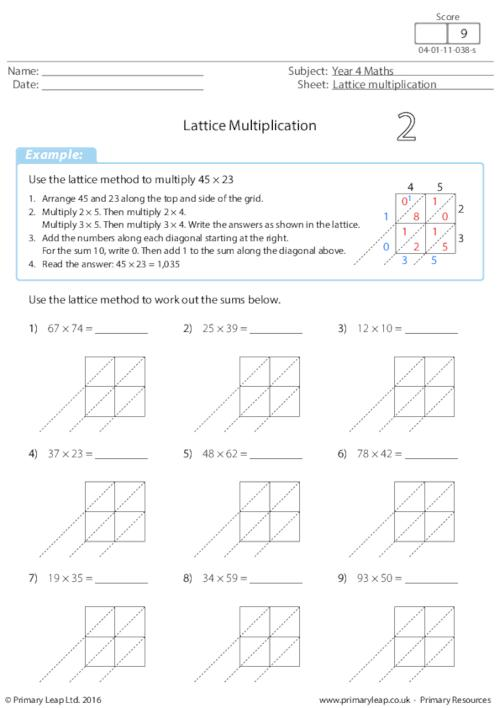### Lattice Multiplication: 2 by 2 digits (1)

Lattice multiplication is a method of multiplying numbers using a grid. This method breaks the multiplication process into smaller steps, which some students may find easier.

Age: 8 - 9
1
2
3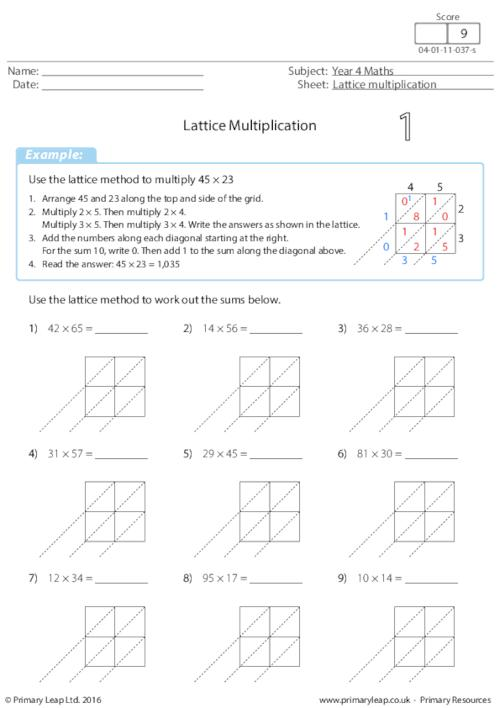### Solving the Equation - Multiplication and division

Maths: Students are asked to work out the value of each letter to complete the sum. There are 14 sums to work out.

Age: 8 - 9
1
2
3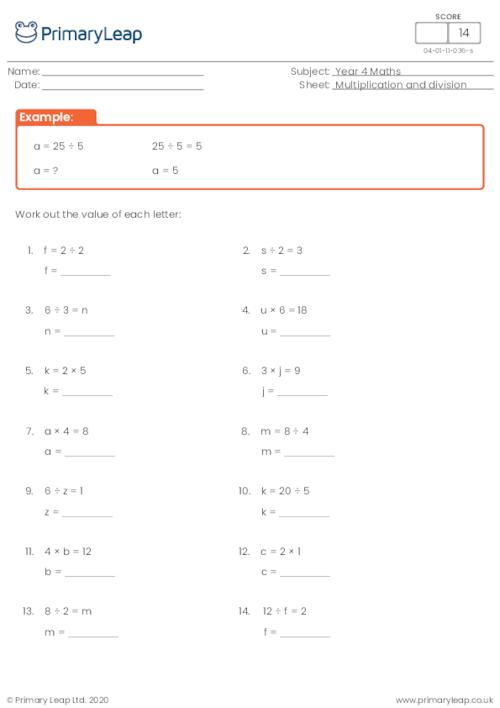### Sequences - Multiples of 6

A multiple of a number is a number which can be divided exactly by that number. 72 can be divided exactly by 6, so 72 is a multiple of 6. Students must complete the multiples of 5 sequences.

Age: 8 - 9
1
2
3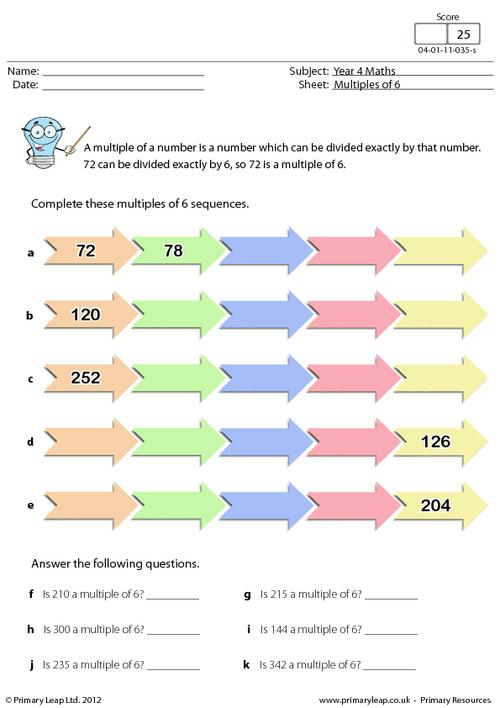### Sequences - Multiples of 5

A multiple of a number is a number which can be divided exactly by that number. 225 can be divided exactly by 5, so 225 is a multiple of 5. Students must complete the multiples of 5 sequences.

Age: 8 - 9
1
2
3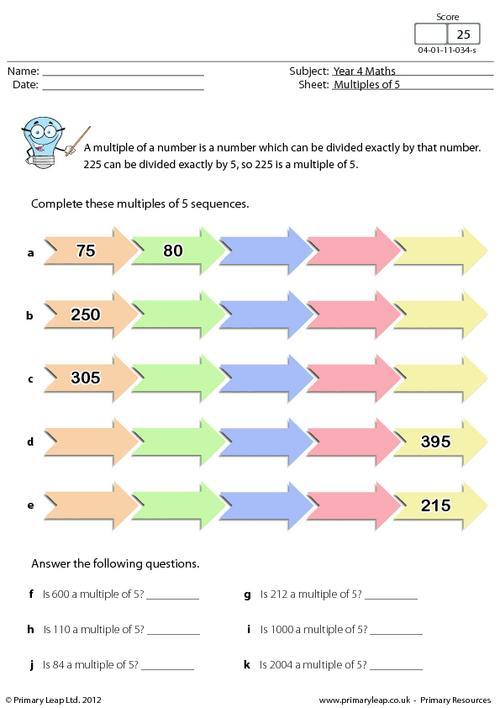### Sequences - Multiples of 4

A multiple of a number is a number which can be divided exactly by that number. 44 can be divided exactly by 4, so 44 is a multiple of 4. Students must complete the multiples of 4 sequences.

Age: 8 - 9
1
2
3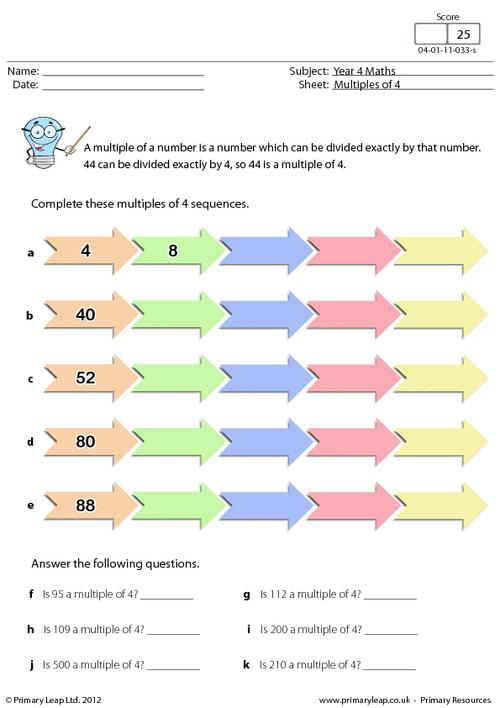### Times table practice

Year 4 Maths - Multiplication. This times table resource is great for learning your times tables. Set yourself a time limit and see how well you do!

Age: 8 - 9
1
2
3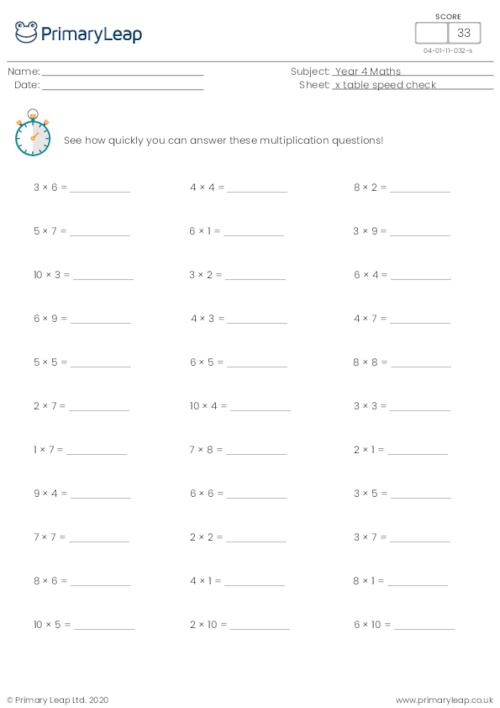### Division

Math: Division - Answer the two sets of division questions.

Age: 8 - 9
1
2
3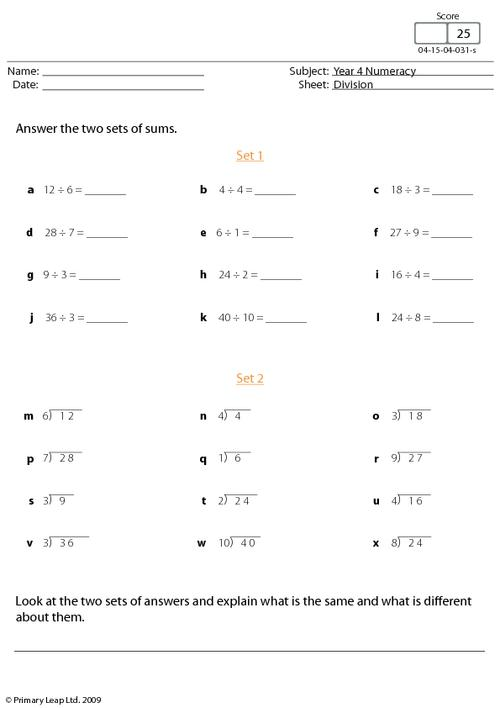### Multiply and divide problems

Students answer the multiplication and division word problems.

Age: 8 - 9
1
2
3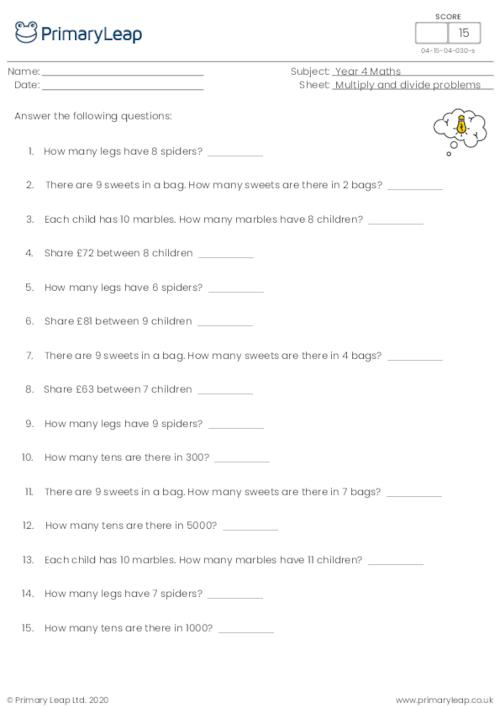### Multiply and divide problems

Age: 8 - 9
1
2
3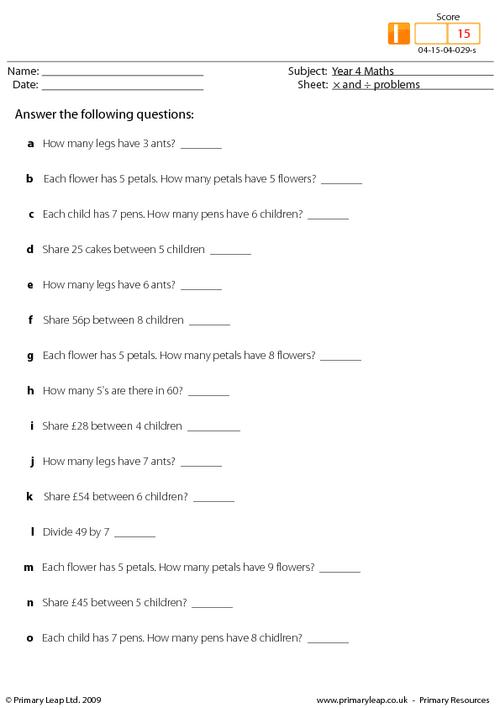### Multiply and divide problems

Age: 8 - 9
1
2
3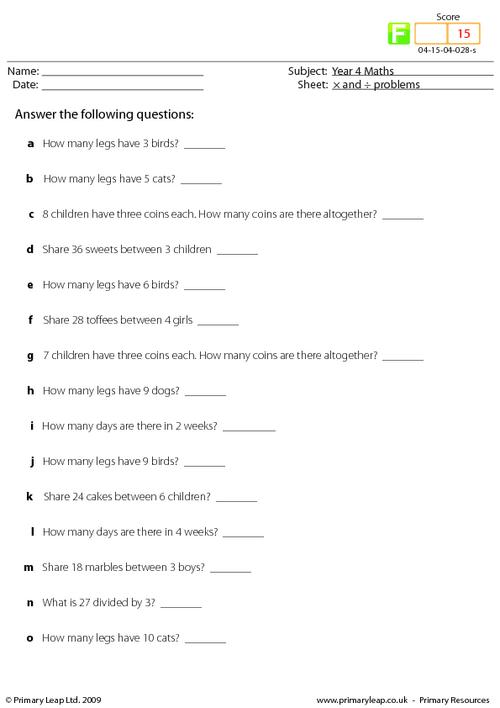### Multiplication facts

Age: 8 - 9
1
2
3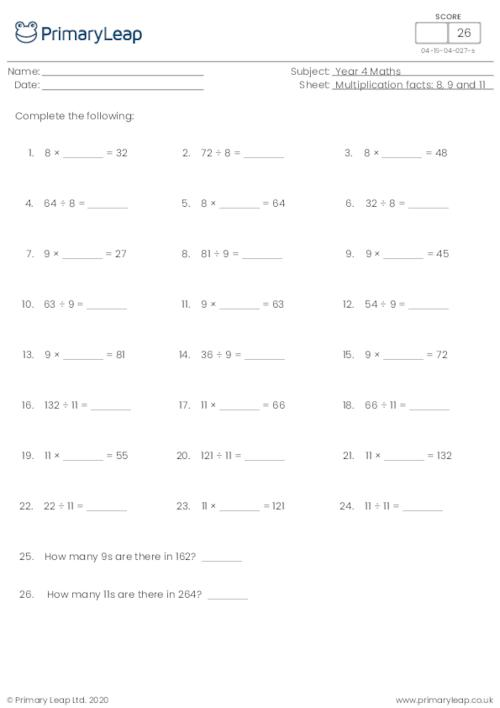### Multiplication facts

Age: 8 - 9
1
2
3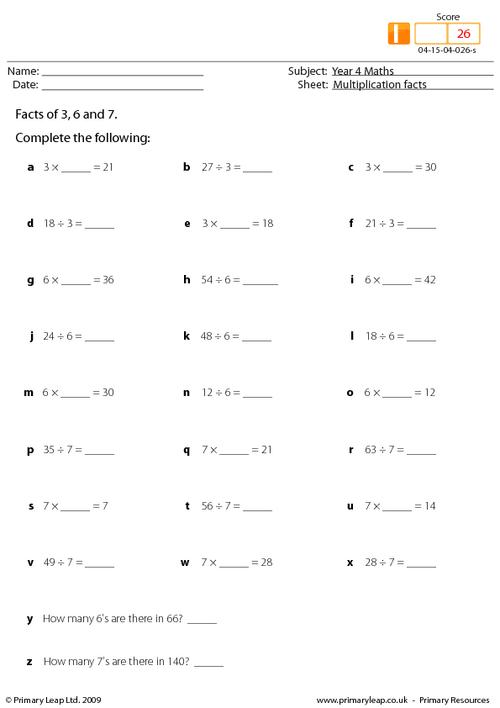### Multiplication facts

Age: 8 - 9
1
2
3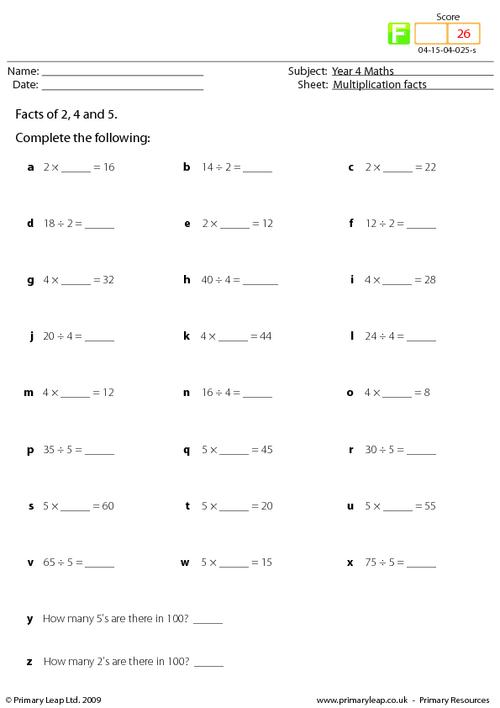### Multiplying using brackets

Age: 8 - 9
1
2
3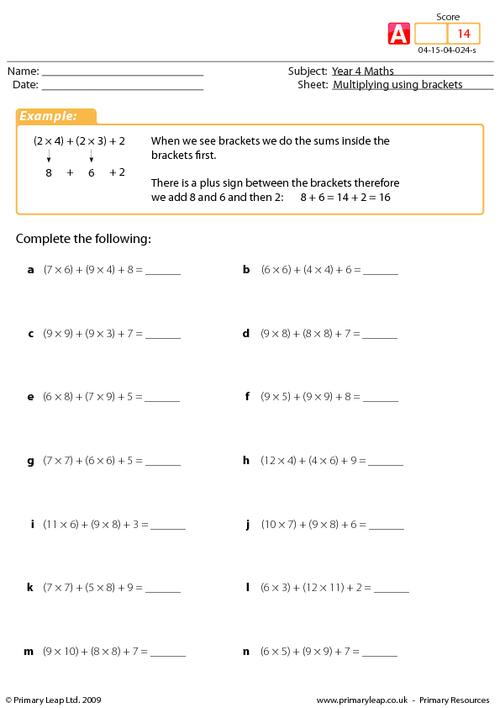### Multiplying using brackets

Multiplying using brackets.

Age: 8 - 9
1
2
3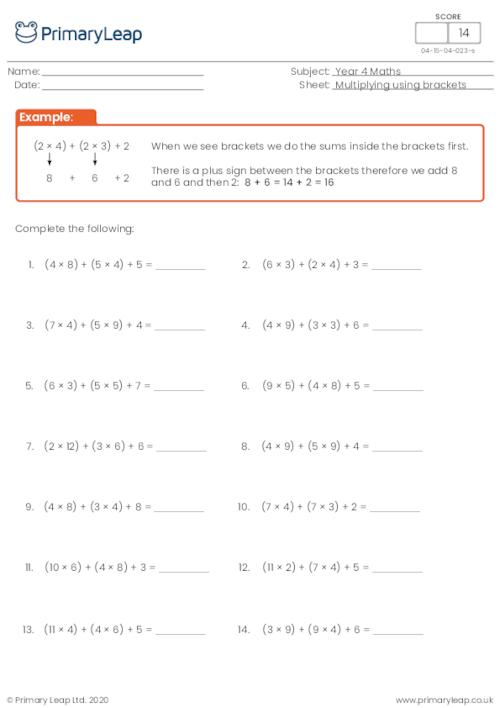### Multiplying using brackets

Age: 8 - 9
1
2
3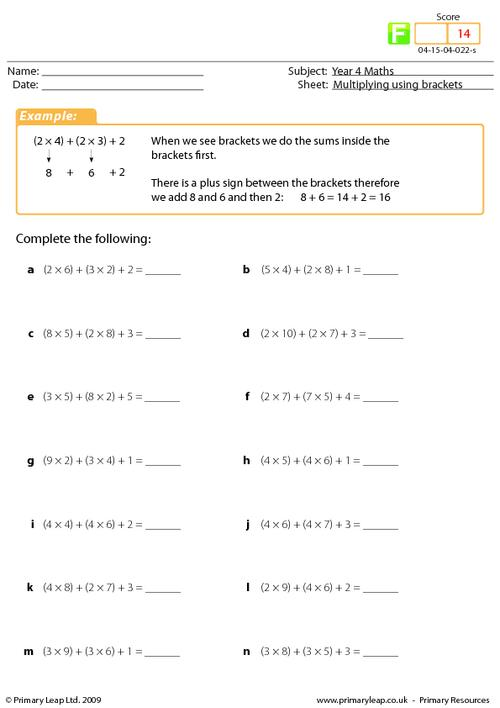### Dividing as fractions

Age: 8 - 9
1
2
3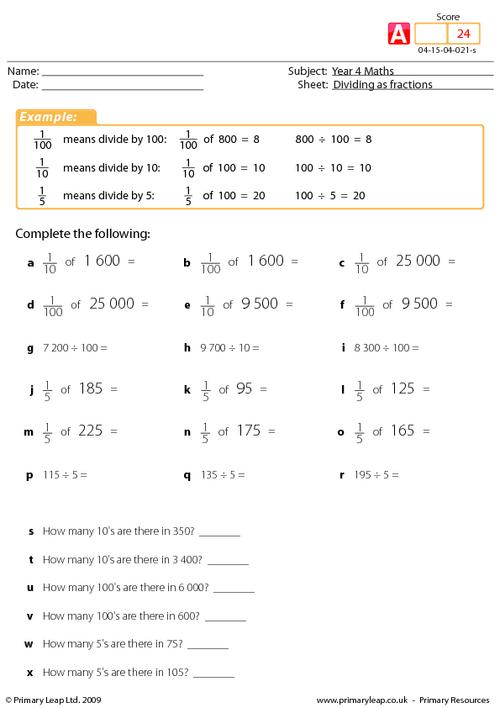### Dividing as fractions

Age: 8 - 9
1
2
3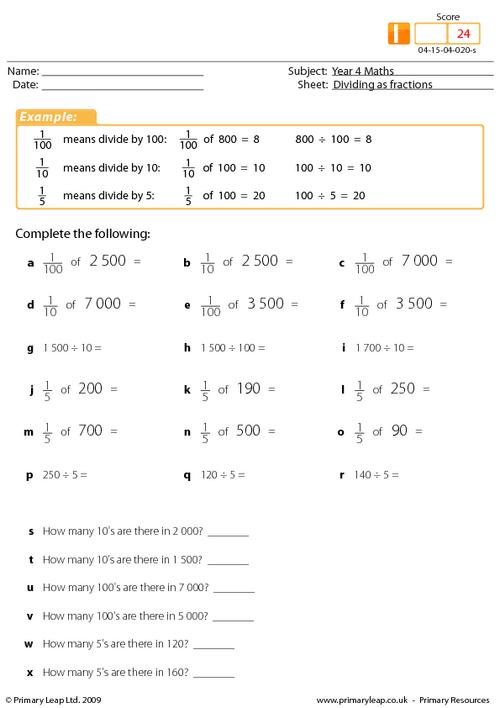### Dividing as fractions

Age: 8 - 9
1
2
3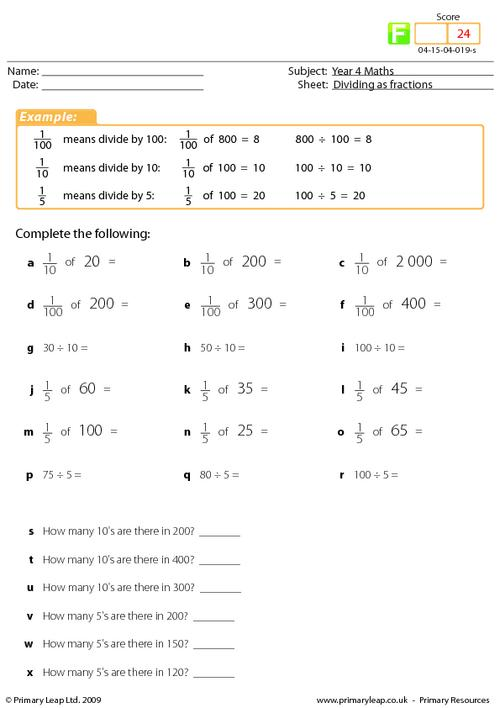### Divide and multiply by 4

Age: 8 - 9
1
2
3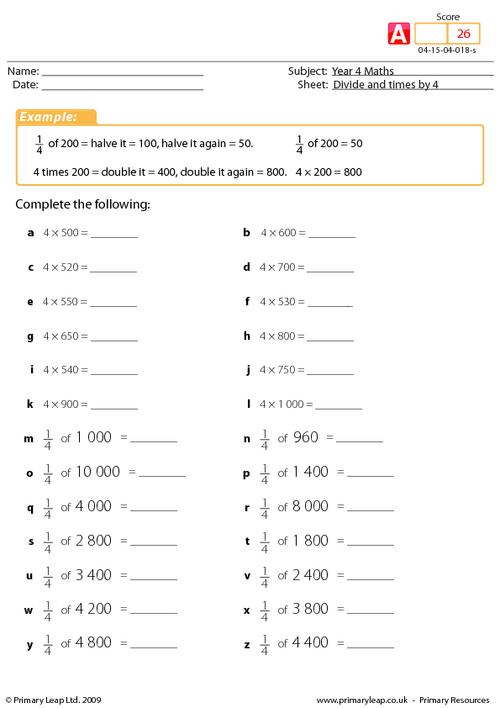### Divide and multiply by 4

Age: 8 - 9
1
2
3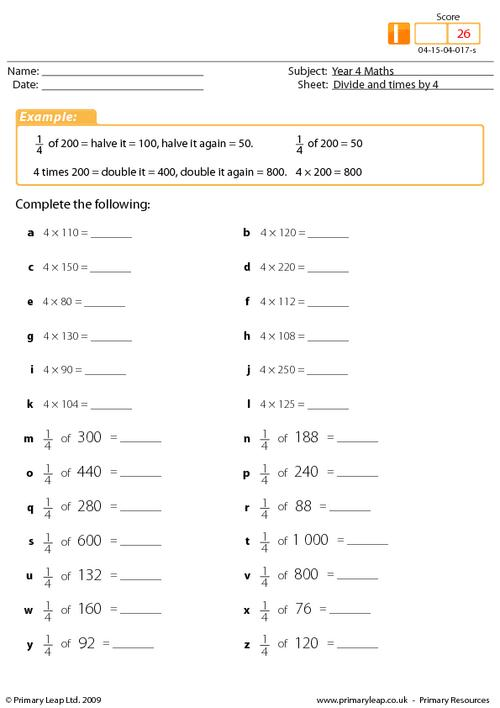### Divide and multiply by 4

Age: 8 - 9
1
2
3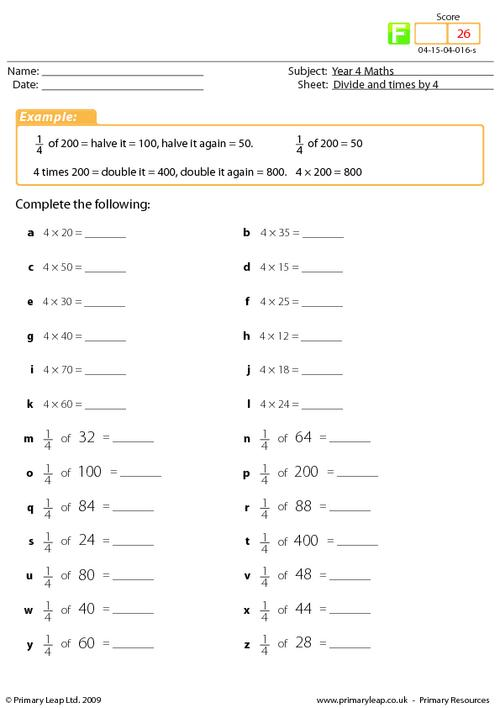### Multiplication squares

Age: 8 - 9
1
2
3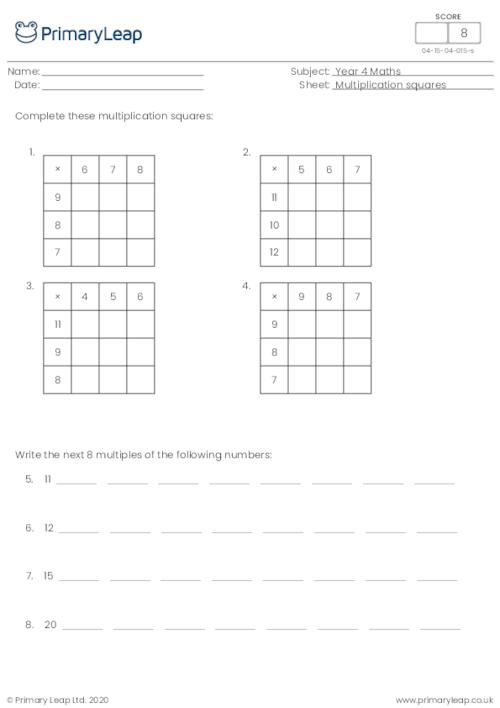### Multiplication squares

Age: 8 - 9
1
2
3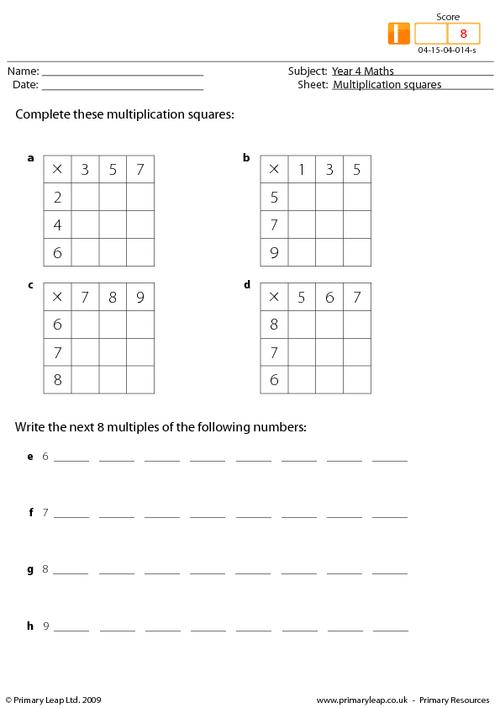### Multiplication squares

Age: 8 - 9
1
2
3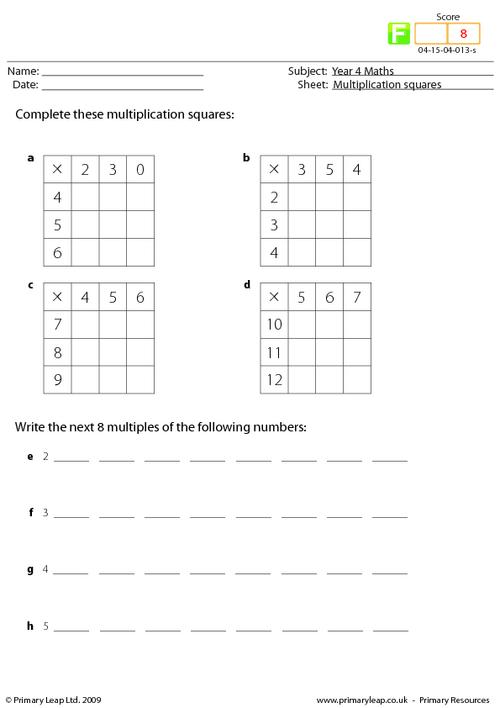### Division

Age: 8 - 9
1
2
3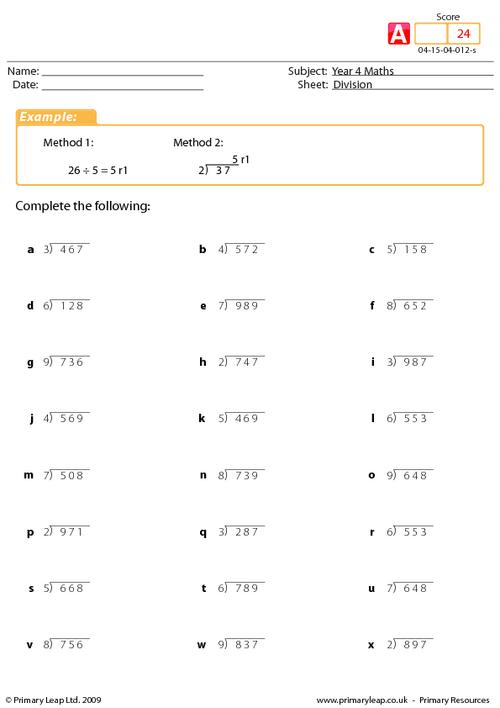### Division

Age: 8 - 9
1
2
3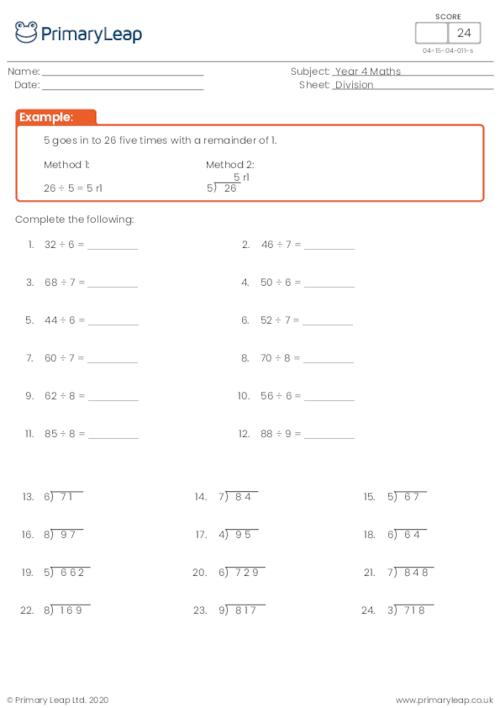### Division

Age: 8 - 9
1
2
3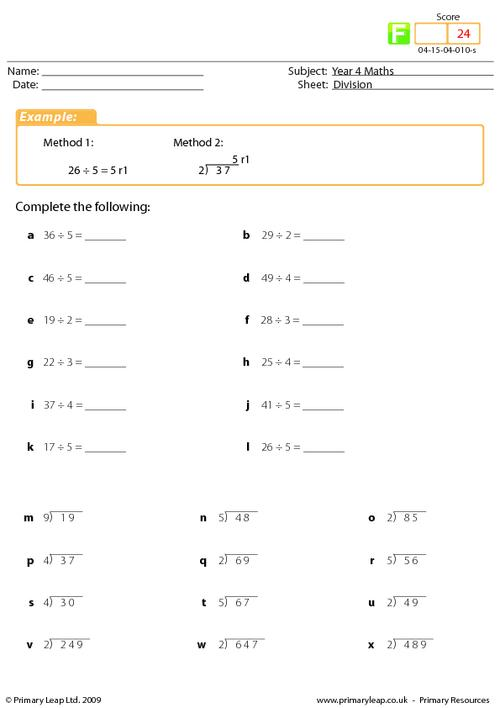### Double or half

Age: 8 - 9
1
2
3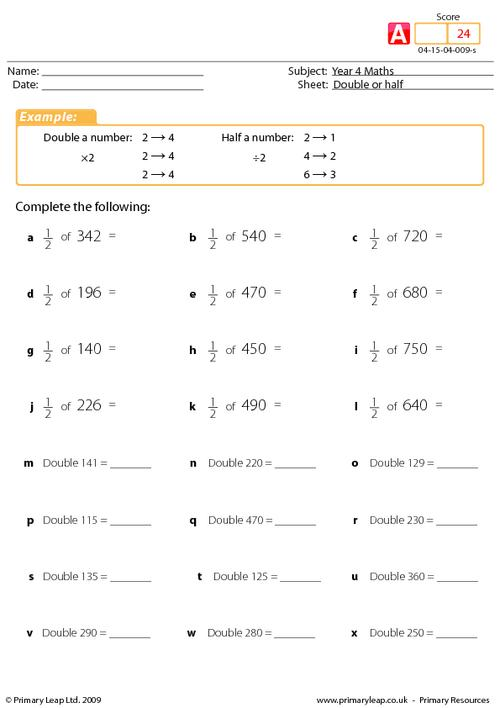### Double or half

Age: 8 - 9
1
2
3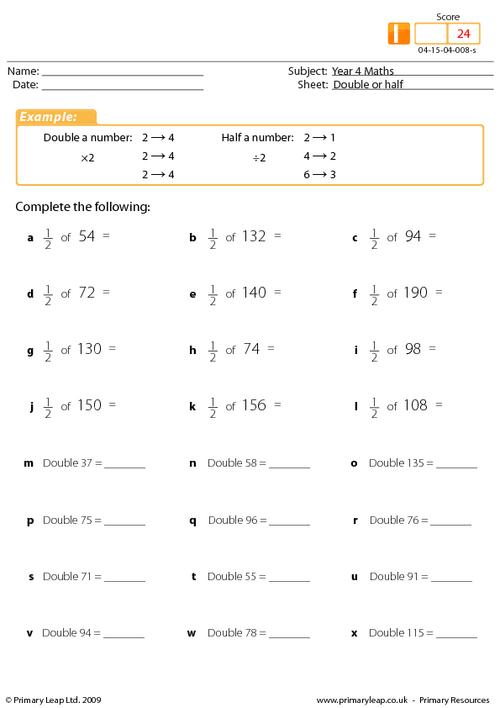### Double or half

Age: 8 - 9
1
2
3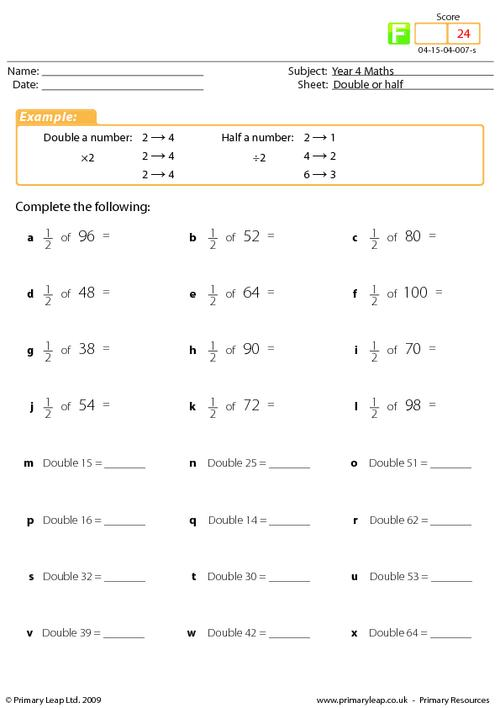### Vertical multiplication

Age: 8 - 9
1
2
3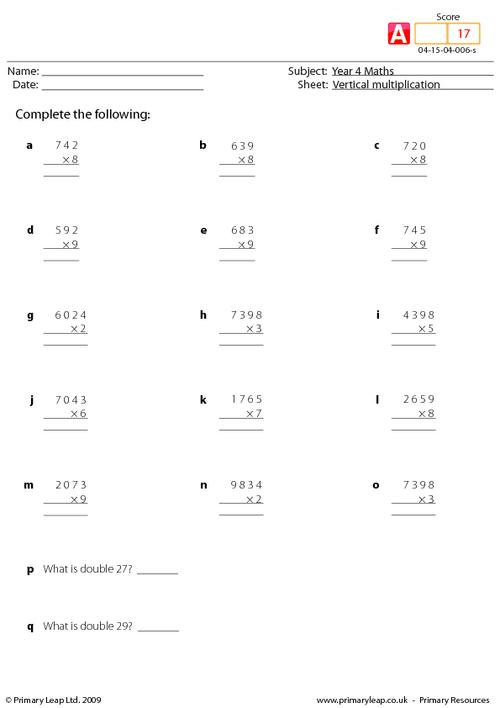### Vertical multiplication

Age: 8 - 9
1
2
3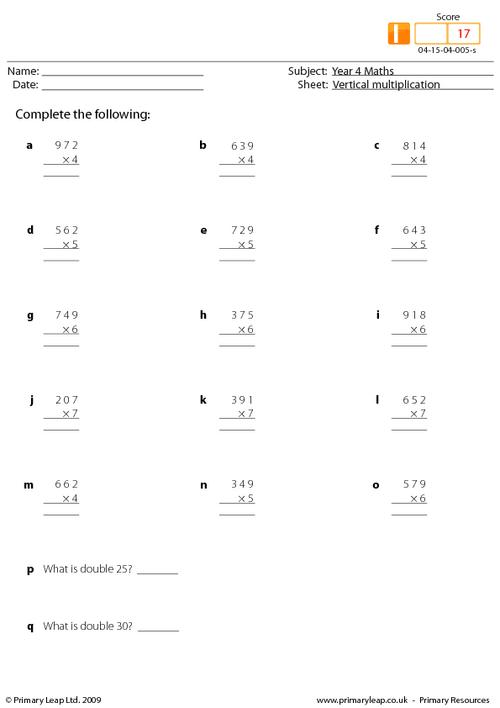### Vertical multiplication

Age: 8 - 9
1
2
3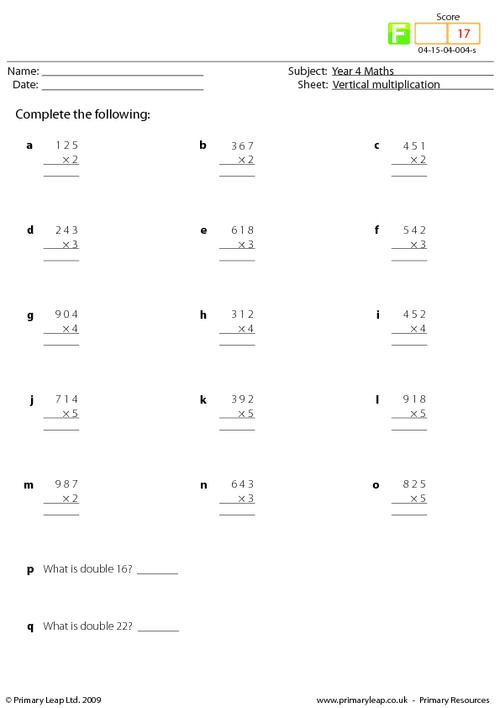### Simple multiplication

Age: 8 - 9
1
2
3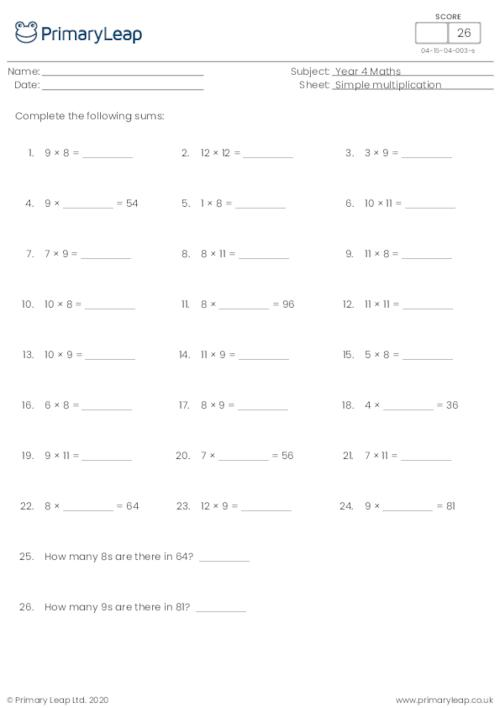### Simple multiplication

Age: 8 - 9
1
2
3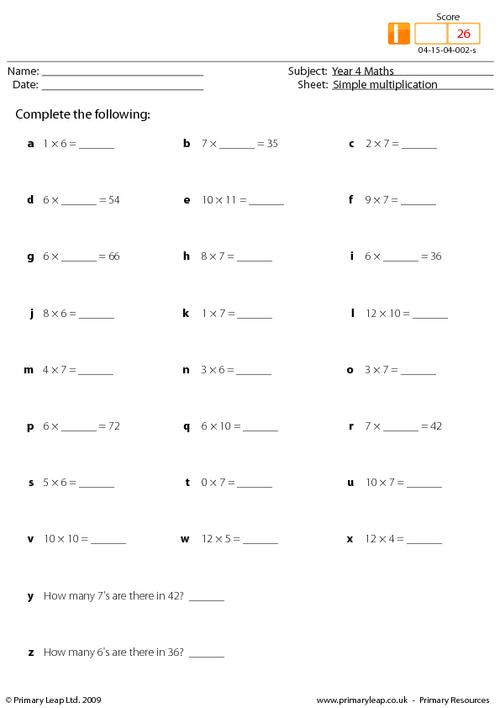### Simple multiplication

Age: 8 - 9
1
2
3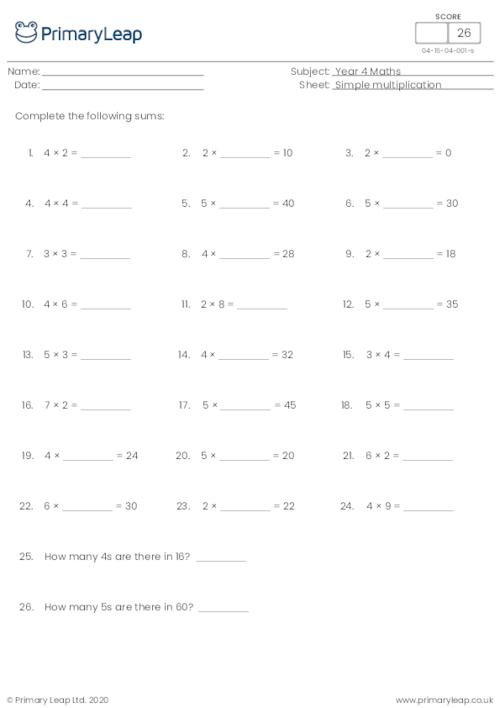of 1## Help your child develop their mental calculation skills with our year 4 maths worksheets

Our fun and engaging year 4 maths activities will help your child develop their mental and written calculation skills using larger positive numbers and fractions. Our maths worksheets will help your child learn to count in steps of 6, 7, 9, 25 and 1000, add and subtract four-digit numbers, multiply three-digit by one-digit numbers, and be able to divide by 10 and 100 to give decimal answers. Don’t worry if some of the methods are new to you, all of our maths worksheets come complete with answer sheets!Learn

One of the best things about printable worksheets is that they make learning fun and easy. Explore our educational resources based on Year 4 Maths and keep your children learning through these fun and engaging exercises.Progress

Our Year 4 Maths printable worksheets are designed for progressive learning. Our engaging content enables a child to learn at their own speed and gain confidence quickly. When we help a child believe in themselves, we empower them for life.Improve

Printable worksheets are a great way to enhance creativity and improve a child’s knowledge. Help your child learn more about Year 4 Maths with this great selection of worksheets designed to help children succeed.

We use cookies to improve your experience on our site and to show you personalized advertising.

To find out more, read our privacy policy and cookie policy.